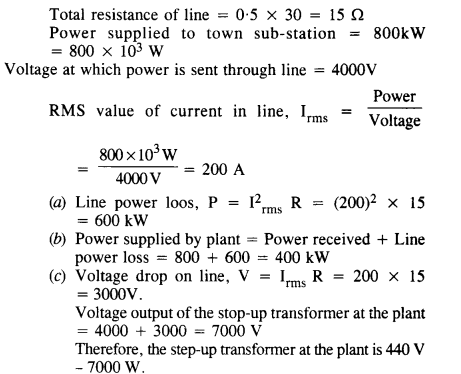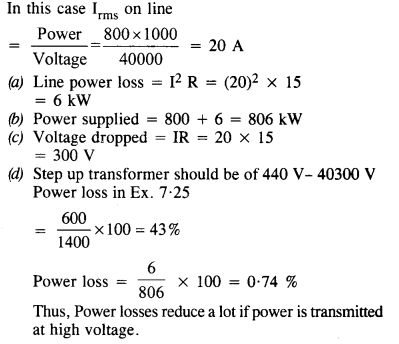## NCERT Solutions for Class 12 Physics Chapter 7 Alternating Current

NCERT Solutions for Class 12 Physics Chapter 7 Alternating Current are part of NCERT Solutions for Class 12 Physics. Here we have given. NCERT Solutions for Class 12 Physics Chapter 7 Alternating Current

 Board CBSE Textbook NCERT Class Class 12 Subject Physics Chapter Chapter 7 Chapter Name Alternating Current Number of Questions Solved 26 Category NCERT Solutions

Question 1.
A 100 Ω resistor is connected to a 220 V, 50 Hz ac supply.
(a) What is the rms value of current in the circuit?
(b) What is the net power consumed over a full cycle?Question 2.
(a) The peak voltage of an a.c. supply is 300 V. What is the rms voltage?
(b) The rms value of current in an ac circuit is 10 A. What is the peak current?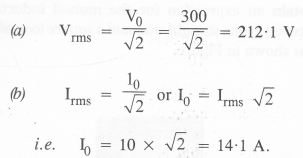Question 3.
A 44 mH inductor is connected to 220 V, 50 Hz ac supply. Determine the rms value of the current in the circuit.
Here, reactance XL = 2 Πv L = 2Π X 50 x 44 x 10-3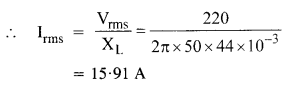Question 4.
A 60 μF capacitor is connected to a 110 V, 60 Hz ac supply. Determine the RMS value of the current in the circuit.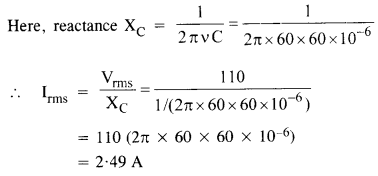Question 5.
In Exercise 7.3 and 7.4, what is the net power absorbed by each circuit over a complete cycle? Explain your answer.
In the case of an ideal inductor or capacitor, there is no power loss.

Question 6.
Obtain the resonant frequency ωr of a series LCR circuit with L =2.0 H, C = 32 μF and R = 10 Ω. What is the Q-value of this circuit?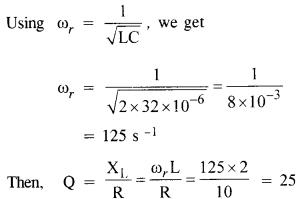Question 7.
A charged 30 μF capacitor is connected to a 27 mil inductor. What is the angular frequency of free oscillations of the circuit ?Question 8.
Suppose the initial charge on the capacitor in Exercise 7.7 is 6 mC. What is the total energy stored in the circuit initially? What is the total energy at later time?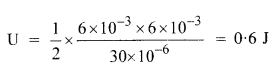At a later time, energy is shared between capacitor and inductor, However, the total energy remains the same, provided there is no loss of energy.

Question 9.
A series LCR circuit with R = 20 Ω, L = 1.5 H and C = 35 μF is connected to a variable-frequency 200 V ac supply. When the frequency of the supply equals the natural frequency of the circuit, what is the average power transferred to the circuit in one complete cycle?
At natural frequencyQuestion 10.
A radio can tune over the frequency range of a portion of the MW broadcast band : (800 kHz to 1200 kHz). If its LC circuit has an effective inductance of 200 pH, what must be the range of its variable capacitor?
[Hint. For tuning, the natural frequency i.e., the frequency of free oscillations for the LC circuit should be equal to the frequency of the radio wave.]Thus, the range of the variable capacitor must be 88 pF to 198 pF.

Question 11.
Figure shows a series LCR circuit connected to a variable frequency 230 V source. L = 5.0 H, C = 80 μF, R = 40 Cl.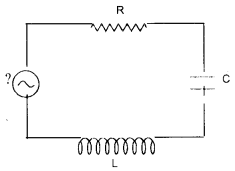(a) Determine the source frequency which drives the circuit in resonance.
(b) Obtain the impedance of the circuit and the amplitude of current at the resonating frequency.
(c) Determine the rms potential drops across the three elements of the circuit. Show that the potential drop across the LC combination is zero at the resonating frequency. (C.B.S.E. 1994, 1998, 2006)
(a) Resonant angular frequency,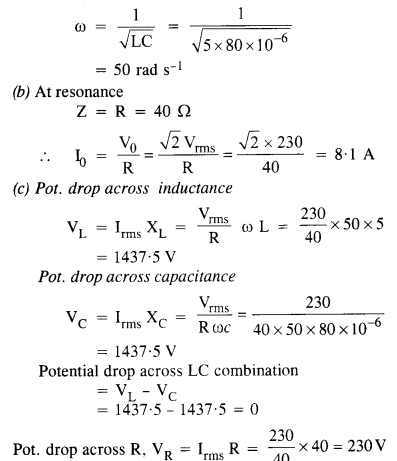Question 12.
An LC circuit contains a 20 mH inductor and a 50 μF capacitor with an initial charge of 10 mC. The resistance of the circuit is negligible. Let the instant the circuit is closed be t = 0.
(a) What is the total energy stored initially? Is it conserved during LC oscillations?
(b) What is the natural frequency of the circuit?
(c) At what time is the energy stored:
(i) completely electrical (i.e., stored in the capacitor)?
(ii) completely magnetic (i.e., stored in the inductor)?
(d) At what times is the total energy shared equally between the inductor and the capacitor?
(e) If a resistor is inserted in the circuit, how much energy is eventually dissipated as heat? (C.B.S.E. Sample Paper 1998)
(a) Total initial energy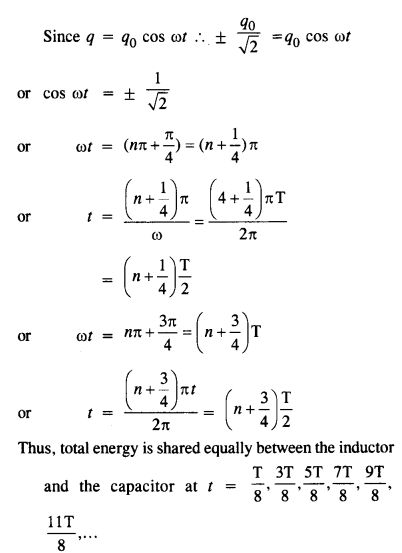(e) Total initial energy of 1 J will be lost as heat due to Joule’s heating effect in the resistor.

Question 13.

A coil of inductance 0.50 H and resistance 100 Ω is connected to a 240 V, 50 Hz ac supply.
(a) What is the maximum current in the coil?
(b) What is the time lag between the voltage maximum and the current maximum?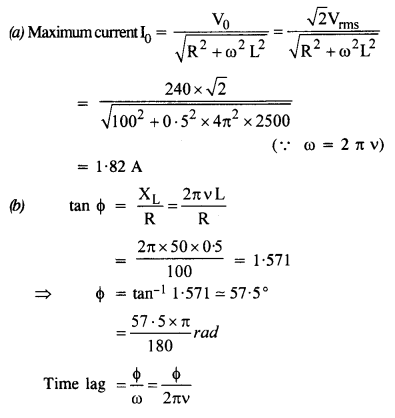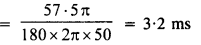Question 14.

Obtain the answers (a) to (b) in Exercise 7.13 if the circuit is connected to a high-frequency supply (240 V, 10 kHz). Hence, explain the statement that at very high frequency, an inductor in a circuit nearly amounts to an open circuit. How does an inductor behave in a dc circuit after the steady-state?
For the given high frequency, ω = 2 Π v = 2 Π x 104 rad s-1
I0, in this case, is too small, so it can be concluded that at high frequencies an inductor behaves as on an open circuit.
In a steady d.c. circuit v = 0, so the inductor acts as a simple conductor.

Question 15.
A 100 μF capacitor in series with a 40 Ω resistance is connected to a 110 V, 60 Hz supply.
(а)    What is the maximum current in the circuit?
(b)  What is the time lag between the current maximum and the voltage maximum?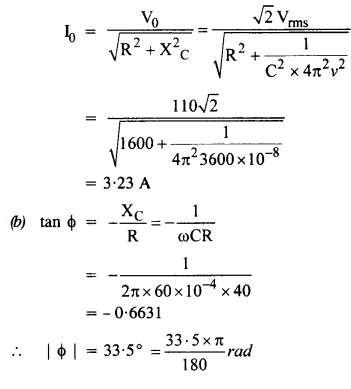Question 16.
Obtain the answers to (a) and (b) in Exercise 7.15 if the circuit is connected to a 110 V, 12 kHz supply. Hence, explain the statement that a capacitor is a conductor at very high frequencies. Compare this behavior with that of a capacitor in a dc circuit after the steady-state.embed=”true”>or n is nearly zero at high frequency. In part (a) C term is negligible at a high frequency so it acts like a resistor. For a steady d. c. we have v like

Question 17.
Keeping the source frequency equal to the resonating frequency of the series LCR circuit, if the three elements. L, C, and R are arranged in parallel, show that the total current in the parallel LCR circuit is minimum at this frequency. Obtain the current rms value in each branch of the circuit for the elements and source specified in Exercise 7.11 for this frequency.
In the case of parallel LCR circuit, impedance is given by,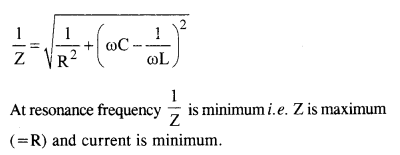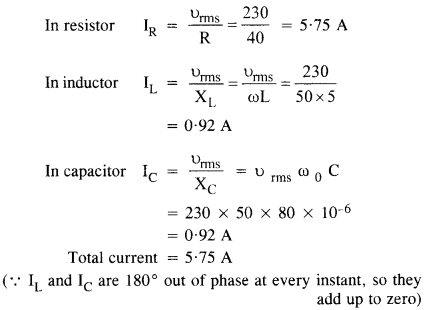Question 18.
A circuit containing an 80 mF inductor and a 60 μF capacitor in series is connected to a 230 V, 50 Hz supply. The resistance of the circuit is negligible.
(a) Obtain the current amplitude and rms values.
(b) Obtain the rms values of potential drops across each element.
(c) What is the average power transferred to the inductor?
(d) What is the average power transferred to the capacitor?
(e) What is the total average power absorbed by the circuit?
[‘Average’ implies ‘averaged over one cycle’.]Question 19.
Suppose the circuit in Exercise 7.18 has a resistance of 15 Ω. Obtain the average power transferred to each element of the circuit, and the total power absorbed.Question 20.
A series LCR circuit with L = 0.12 H, C = 480 nF, R = 23 Ω is connected to a 230 V variable frequency supply.
(a) What is the source frequency for which current amplitude is maximum. Obtain this maximum value.
(b) What is the source frequency for which the average power absorbed by the circuit is maximum. Obtain the value of this maximum power.
(c) For which frequencies of the source are the power transferred to the circuit half the power at resonant frequency? What is the current amplitude at these frequencies?
(d) What is the Q-factor of the given circuit? (C.B.S.E. 1992 )Question 21.
Obtain the resonant frequency and Q-factor of a series LCR circuit with L = 3.0 H, C = 27 μF, and R = 7.4 Ω. It is desired to improve the sharpness of the resonance of the circuit by reducing its ‘full width at half maximum’ by a factor of 2. Suggest a suitable way.Question 22.
(a) In any ac circuit, is then applied instantaneous voltage equal to the algebraic sum of the instantaneous voltages across the series elements of the circuit? Is the same true for rms voltage?
(b) A capacitor is used in the primary circuit of an induction coil.
(c) An applied voltage signal consists of a superposition of a dc voltage and an ac voltage of high frequency. The circuit consists of an inductor and a capacitor in series. Show that the dc signal will appear across C and the ac signal across L.
(d) A choke coil in series with a lamp is connected to a dc line. The lamp is seen to shine brightly. Insertion of an iron core in the choke causes no change in the lamp’s brightness. Predict the corresponding observations if the connection is to an ac line.
(e) Why is choke coil needed in the use of fluorescent tubes with ac mains? Why can we not use an ordinary resistor instead of the choke coil?
(a) Yes, the applied instantaneous voltage is equal to the algebraic sum of the instantaneous voltages across the series elements of the circuit. It is because voltages across different elements are not in phase.
It is not true for rms voltages. It is because rms voltages across different elements are not in phase with each other.

(b) At the break, a large induced emf is produced. In case the capacitor is not connected, sparking will take place. But when the capacitor is used, the large induced emf produced at break is used up in charging the capacitor and no sparking takes place.

(c)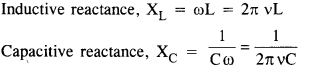For d.c., XL = 0, therefore, XL = 0 and Xc = oo. Hence, d.c. signal appears across capacitor.For high frequency a.c., XL → High and Xc → 0. Hence, a.c. signal appears across inductor.

(d) When a choke coil in series with a lamp is connected to a d.c. line, L has no effect on the steady value of the current. Therefore, the brightness of the lamp is not affected by the insertion of the iron core in the choke. On a.c. line, the lamp will shine dimly due to the impedance of the choke coil. The brightness of the lamp will further go dim on the insertion of an iron core, which increases the impedance of the choke coil.

(e) The choke coil is used to reduce the current. As its power factor is zero, it reduces the current without wasting the power. If an ordinary resistor is used instead of a choke coil, ft will waste power in the form of heat.

Question 23.
A power transmission line feeds input power at 2300 V to a step-down transformer with its primary windings having 4000 turns. What should be the number of turns in the secondary in order to get output power at 230 V? (C.B.S.E. 1997)Question 24.
At a hydroelectric power plant, the water pressure head is at a height of 300 m and the water flow available is 100 m3s-1. If the turbine generator efficiency is 60%, estimate the electric power available from the plant (g = 9.8 ms-2).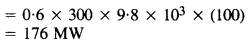Question 25.
A small town with a demand of 800 kW of electric power at 220 V is situated 15 km away from an electric plant generating power at 440 V. The resistance of the two wirelines carrying power is 0.5 Q per km. The town gets power from the line through a 4000-220 V step-down transformer at a sub-station in the town.
(a) Estimate the line power loss in the form of heat.
(b) How much power must the plant supply, assuming there is negligible power loss due to leakage?
(c) Characterise the step-up transformer at the plant.(C.B.S.E. Sample Paper 2003)